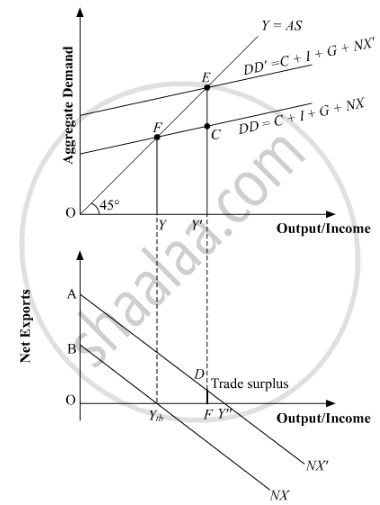# Answer the Following Question. "Indian Rupee (₹) Plunged to an All-time Low of ₹ 74.48 Against the Us Dollar ($)". − the Economic Times in Light of the Above Report, Discuss the Impact of the - Economics Advertisement Advertisement Advertisement Answer in Brief Answer the following question. "Indian Rupee (₹) plunged to an all-time low of ₹ 74.48 against the US Dollar ($)".
− The Economic Times
In light of the above report, discuss the impact of the situation on Indian Imports.

#### Solution

Indian Rupee is depreciating against the US Dollar since it is given that " Indian Rupee (₹) plunged to an all-time low of ₹ 74.48 against the US Dollar (\$)".A high exchange rate makes the imports more expensive. Consequently, a rise in the exchange rate implies a reduction in the demand for imports and vice-versa.
When imports fall, net exports (Exports - Imports) of a country rise. The given figure explains this process as follows:
Suppose the initial equilibrium income is given by Ye that corresponds to a trade balance equal to Ytb. With the rise in the net export demand, the aggregate demand curve DD shifts upwards to DD' such that the new equilibrium is established at point E' and the equilibrium income rises to Y'.
In the lower panel due to the fall in the imports, the net export rises and the net export curve shifts upwards from NX to NX'.At the new level of income, the net exports is represented by the vertical distance AE' which are necessarily positive (because the total demand curve DD' lies above the aggregate demand curve AD). Thus, with a fall in imports, there is a trade surplus. This trade surplus is represented in the lower panel by the vertical length DF.Concept: Determination of Equilibrium Income in the Short Run - Effect of an Autonomous Change in Aggregate Demand on Income and Output
Is there an error in this question or solution?
2018-2019 (March) 58/1/1

Share